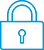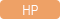cancel
Showing results for
Search instead for
Did you mean:It has been a while since anyone has replied. Simply ask a new question if you would like to start the discussion again.New member
5 2 0 0
Message 1 of 4
1,429
Flag Post

Solved!

# Input Multivariable Fucntions as a VectorHP Recommended
HP Prime

Hello,

I'm creating a program for the Hp Prime in which the user has to enter a multiple variable function as a vector, something like this:

[x^2+2*y*z-3*z];

I'm using command INPUT.

However, there's always a syntax error message when I input that kind of vector. Is there a way to do this?

Here, this is a part of the program I'm doing:

EXPORT Multfunc()

BEGIN

LOCAL FX1;

FX1:=;

INPUT(FX1,"INPUT FUNCTION","f=");

PRINT(FX1);

END;

Tags (1)
3 REPLIES 3Level 6
252 251 42 77
Message 2 of 4
Flag PostHP Recommended

Hello,

The main issue here is that INPUT is a home function and that you are trying to use it for CAS type data. This kinds of breaks things...

You could enter the data as a list of functions, even as a list of cas functions, but it would mean that the user would have to enter somethign like:

cas("{x^2, y^2}") which is much less intuitive than entering just the raw vector.

This is, unfortunately the best I can think of at this point in time.

You could also request the entry to be done as a string (use {{FX1, }} for the variable definition. This would result in a string in the variable which you can then manually parse using the CAS. you loose any type of "during input syntax check", but it might allow you to make your program work as you intend.

Cyrille

I am an HP Employee
Tags (1)New member
5 2 0 0
Message 3 of 4
Flag PostHP Recommended

Thanks for the information, I'll try to do what you say. However, I' ve already made another program (CAS program) and I some problems with it. I have posted another question about it, I hope you could help me.Level 6
305 297 32 76
Message 4 of 4
Flag PostHP Recommended

Hello dj2631,

You want to use symbolic variables x,y,z which can not be used in Home mode.

The INPUT command can only be used in a non-CAS program, which is run in Home mode, so this program cannot use them either.

What you can do is entering the vector in a string: "[x^2+2*y*z-3*z]" because a string can contain any content you like.

In order to enter a string you have to use the syntax:

`INPUT({{FX1,}})`

Note the double curly brackets.

 means that the input is of type string. (see the Help for TYPE)

The quote signs are hidden in the input box but in reality they are present.

So when you enter [x^2+2*y*z-3*z],

FX1 will be the string "[x^2+2*y*z-3*z]"

This first program you only need for the INPUT command.

Now you write a second CAS program.

You call this program in your first program with argument your string.

Buy means of the expr command you convert the string

"[x^2+2*y*z-3*z]" into the vector

[x^2+2*y*z-3*z]

If you like you can create a function by first taking the first element of the vector, which is x^2+2*y*z-3*z, and next write:

f(x,y,z):=x^2+2*y*z-3*z

and you can print this.

This could be your program:

```CASMultfunc(); //declaration of cas function at beginning

EXPORT Multfunc()
BEGIN
LOCAL FX1;
PRINT();
INPUT({{FX1,}});
CASMultfunc(FX1);
END;

#cas
CASMultfunc(a):=
BEGIN
a:=expr(a);a:=a(1);
f(x,y,z):=a;
PRINT(f(x,y,z));
END;
#end

```

† The opinions expressed above are the personal opinions of the authors, not of HP. By using this site, you accept the Terms of Use and Rules of Participation